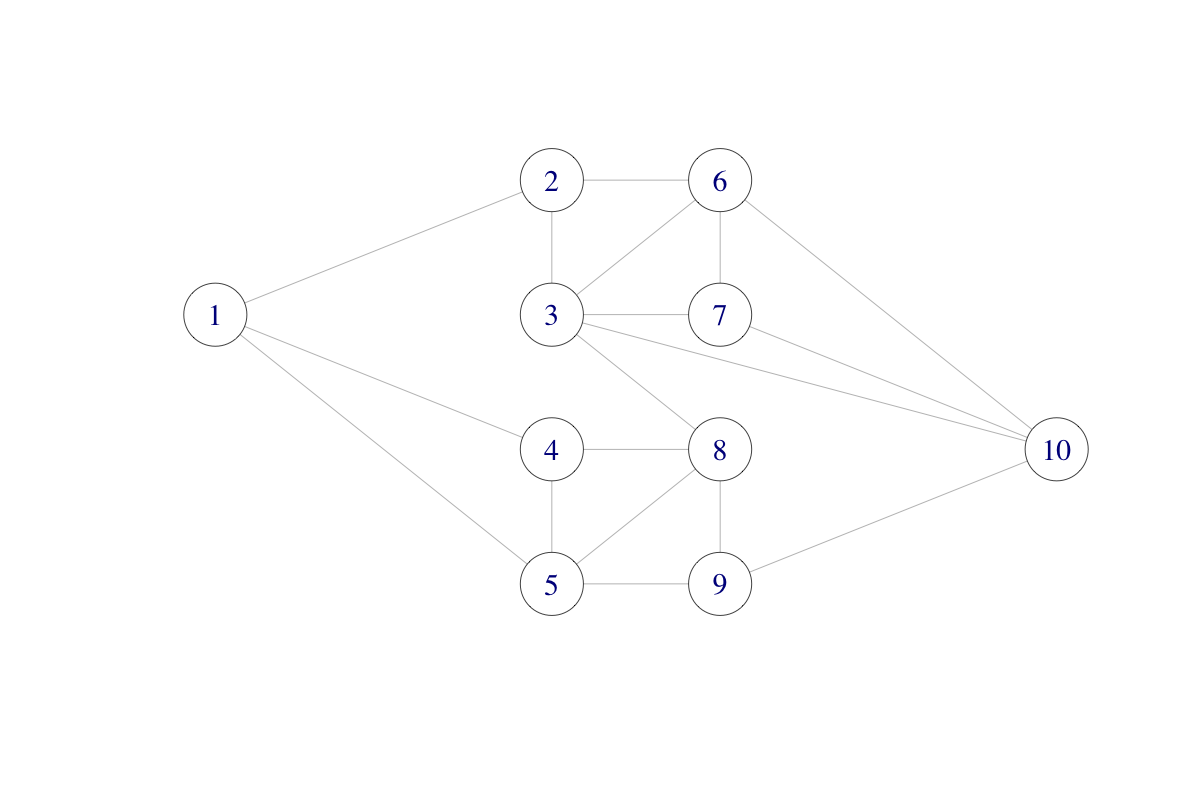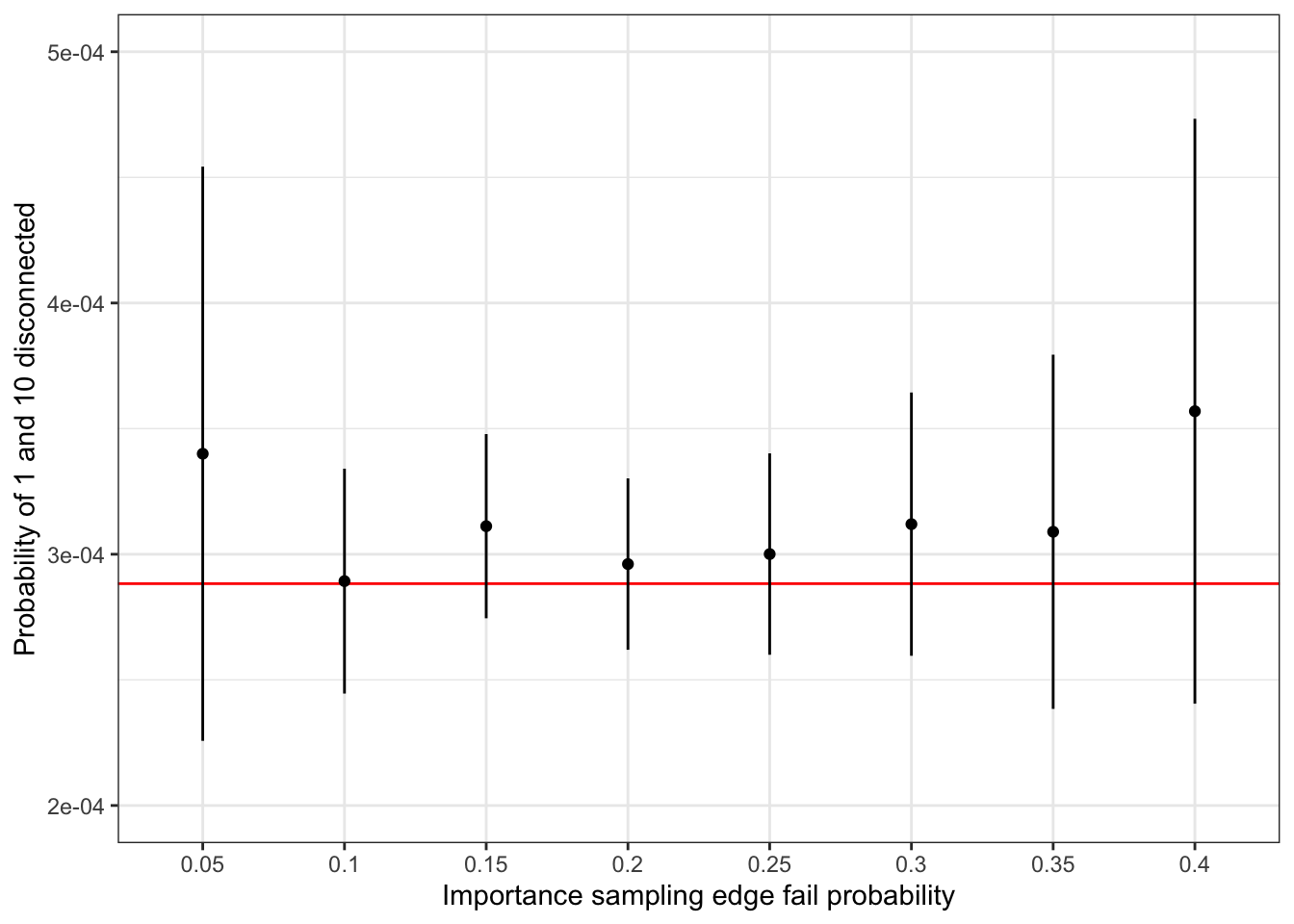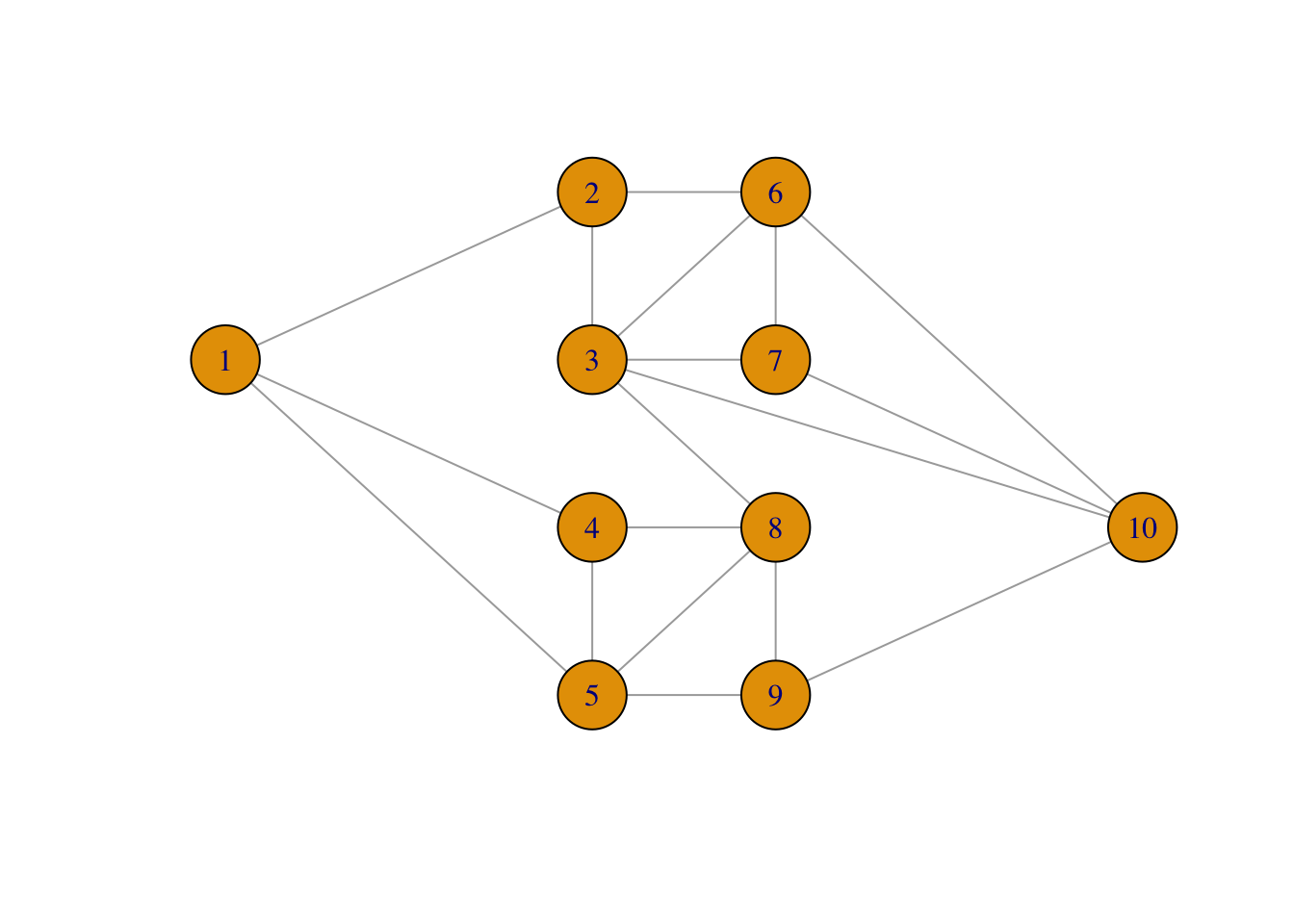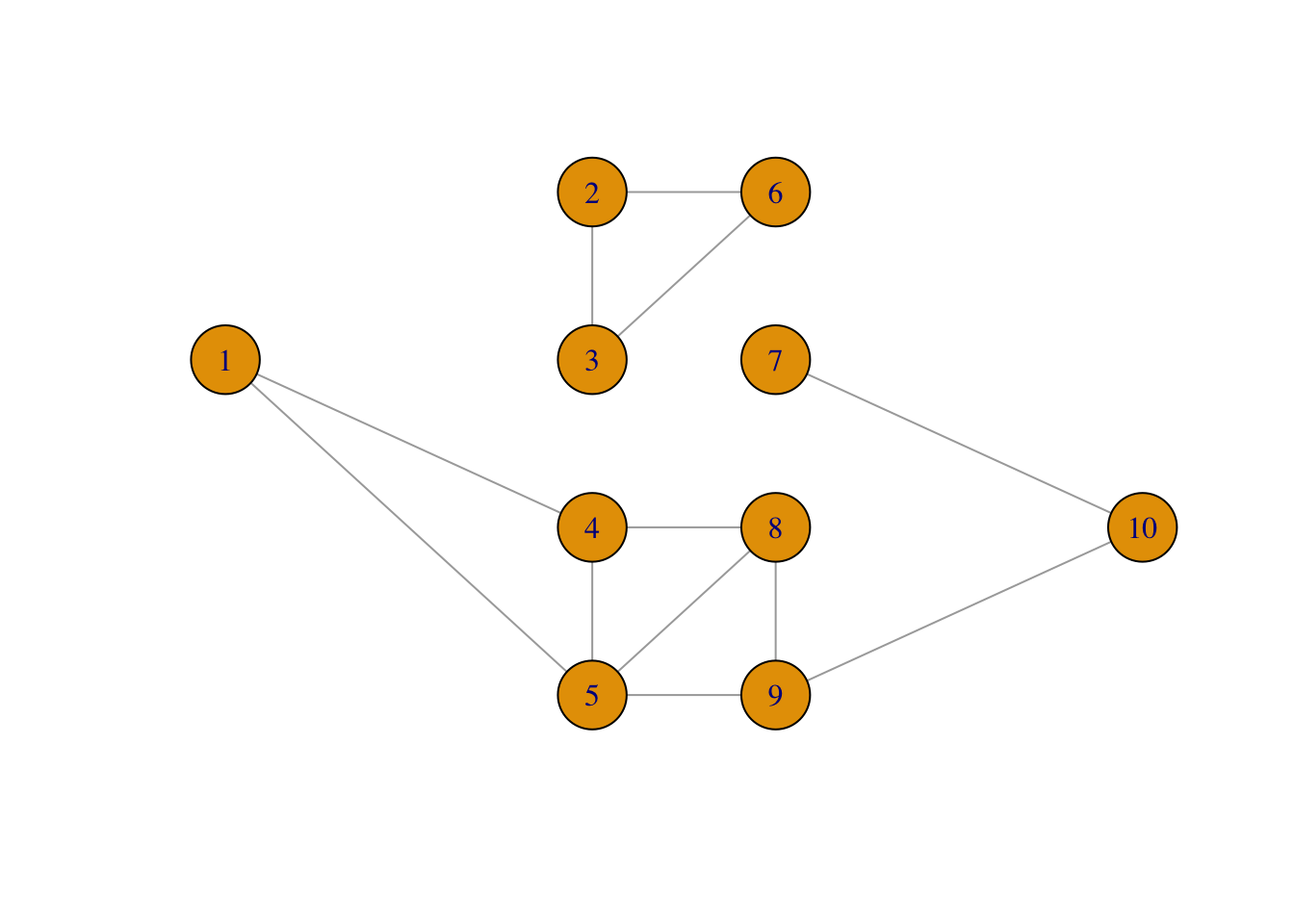## 5.3 Network failure

In this section we consider a more serious application of importance sampling. Though still a toy example, where we can find an exact solution, the example illustrates well the type of application where we want to approximate a small probability using a Monte Carlo average. Importance sampling can then increase the probability of the rare event and as a result make the variance of the Monte Carlo average smaller.

We will consider the following network consisting of ten nodes and with some of the nodes connected.The network could be a computer network with ten computers. The different connections (edges) may “fail” independently with probability $$p$$, and we ask the question: what is the probability that node 1 and node 10 are disconnected?

We can answer this question by computing an integral of an indicator function, that is, by computing the sum $\mu = \sum_{x \in \{0,1\}^{18}} 1_B(x) f_p(x)$ where $$f_p(x)$$ is the point probability of $$x$$, with $$x$$ representing which of the 18 edges in the graph that fail, and $$B$$ represents the set of edges where node 1 and node 10 are disconnected. By simulating edge failures we can approximate the sum as a Monte Carlo average.

The network of nodes can be represented as a graph adjacency matrix $$A$$ such that $$A_{ij} = 1$$ if and only if there is an edge between $$i$$ and $$j$$ (and $$A_{ij} = 0$$ otherwise).

A  # Graph adjacency matrix
##       [,1] [,2] [,3] [,4] [,5] [,6] [,7] [,8] [,9] [,10]
##  [1,]    0    1    0    1    1    0    0    0    0     0
##  [2,]    1    0    1    0    0    1    0    0    0     0
##  [3,]    0    1    0    0    0    1    1    1    0     1
##  [4,]    1    0    0    0    1    0    0    1    0     0
##  [5,]    1    0    0    1    0    0    0    1    1     0
##  [6,]    0    1    1    0    0    0    1    0    0     1
##  [7,]    0    0    1    0    0    1    0    0    0     1
##  [8,]    0    0    1    1    1    0    0    0    1     0
##  [9,]    0    0    0    0    1    0    0    1    0     1
## [10,]    0    0    1    0    0    1    1    0    1     0

To compute the probability that 1 and 10 are disconnected by Monte Carlo integration, we need to sample (sub)graphs by randomly removing some of the edges. This is implemented using the upper triangular part of the (symmetric) adjacency matrix.

sim_net <- function(Aup, p) {
ones <- which(Aup == 1)
Aup[ones] <- sample(
c(0, 1),
length(ones),
replace = TRUE,
prob = c(p, 1 - p)
)
Aup
}

The core of the implementation above uses the sample() function, which can sample with replacement from the set $$\{0, 1\}$$. The vector ones contains indices of the (upper triangular part of the) adjacency matrix containing a 1, and these positions are replaced by the sampled values before the matrix is returned.

It is fairly fast to sample even a large number of random graphs this way.

Aup <- A
Aup[lower.tri(Aup)] <- 0
system.time(replicate(1e5, {sim_net(Aup, 0.5); NULL}))
##    user  system elapsed
##   1.396   0.094   1.506

The second function we implement checks network connectivity based on the upper triangular part of the adjacency matrix. It relies on the fact that there is a path from node 1 to node 10 consisting of $$k$$ edges if and only if $$(A^k)_{1,10} > 0$$. We see directly that such a path needs to consist of at least $$k = 3$$ edges. Also, we don’t need to check paths with more than $$k = 9$$ edges as they will contain the one node multiple times and can thus be shortened.

discon <- function(Aup) {
A <- Aup + t(Aup)
i <- 3
Apow <- A %*% A %*% A # A%^%3
while(Apow[1, 10] == 0 & i < 9) {
Apow <- Apow %*% A
i <- i + 1
}
Apow[1, 10] == 0  # TRUE if nodes 1 and 10 not connected
}

We then obtain the following estimate of the probability of nodes 1 and 10 being disconnected using Monte Carlo integration.

seed <- 27092016
set.seed(seed)
n <- 1e5
tmp <- replicate(n, discon(sim_net(Aup, 0.05)))
mu_hat <- mean(tmp)

As this is a random approximation, we should report not only the Monte Carlo estimate but also the confidence interval. Since the estimate is an average of 0-1-variables, we can estimate the variance, $$\sigma^2$$, of the individual terms using that $$\sigma^2 = \mu (1 - \mu)$$. We could just as well have used the empirical variance, which would give almost the same numerical value as $$\hat{\mu} (1 - \hat{\mu})$$, but we use the latter estimator to illustrate that any (good) estimator of $$\sigma^2$$ can be used when estimating the asymptotic variance.

mu_hat + 1.96 * sqrt(mu_hat * (1 - mu_hat) / n) * c(-1, 0, 1)
##  0.000226 0.000340 0.000454

The estimated probability is low and only in about 1 of 3000 simulated graphs will node 1 and 10 be disconnected. This suggests that importance sampling can be useful if we sample from a probability distribution with a larger probability of edge failure.

To implement importance sampling we note that the point probabilities (the density w.r.t. counting measure) for sampling the 18 independent 0-1-variables $$x = (x_1, \ldots, x_{18})$$ with $$P(X_i = 0) = p$$ is

$f_p(x) = p^{18 - s} (1- p)^{s}$

where $$s = \sum_{i=1}^{18} x_i$$. In the implementation, weights are computed that correspond to using probability $$p_0$$ (with density $$g = f_{p_0}$$) instead of $$p$$, and the weights are only computed if node 1 and 10 are disconnected.

weights <- function(Aup, Aup0, p0, p) {
w <- discon(Aup0)
if (w) {
s <- sum(Aup0)
w <- (p / p0)^18 * (p0 * (1 - p) / (p * (1 - p0)))^s
}
as.numeric(w)
}

The implementation uses the formula

$w(x) = \frac{f_p(x)}{f_{p_0}(x)} = \frac{p^{18 - s} (1- p)^{s}}{p_0^{18 - s} (1- p_0)^{s}} = \left(\frac{p}{p_0}\right)^{18} \left(\frac{p_0 (1- p)}{p (1- p_0)}\right)^s.$

The importance sampling estimate of $$\mu$$ is then computed.

set.seed(seed)
tmp <- replicate(n, weights(Aup, sim_net(Aup, 0.2), 0.2, 0.05))
mu_hat_IS <- mean(tmp)

And we obtain the following confidence interval using the empirical variance estimate $$\hat{\sigma}^2$$.

mu_hat_IS + 1.96 * sd(tmp) / sqrt(n) * c(-1, 0, 1)
##  0.000262 0.000296 0.000330

The ratio of estimated variances for the plain Monte Carlo estimate and the importance sampling estimate is

mu_hat * (1 - mu_hat) / var(tmp)
##  11.22476

Thus we need around 11 times more samples if using plain Monte Carlo integration when compared to importance sampling to obtain the same precision. A benchmark will show that the extra computing time for importance sampling is small compared to the reduction of variance. It is therefore worth the coding effort if used repeatedly, but not if it is a one-off computation.

The graph is, in fact, small enough for complete enumeration and thus the computation of an exact solution. There are $$2^{18} = 262,144$$ different networks with any number of the edges failing. To systematically walk through all possible combinations of edges failing, we use the function intToBits that converts an integer to its binary representation for integers from 0 to 262,143. This is a quick and convenient way of representing all the different fail and non-fail combinations for the edges.

ones <- which(Aup == 1)
Atmp <- Aup
p <- 0.05
prob <- numeric(2^18)
for(i in 0:(2^18 - 1)) {
on <- as.numeric(intToBits(i)[1:18])
Atmp[ones] <- on
if (discon(Atmp)) {
s <- sum(on)
prob[i + 1] <- p^(18 - s) * (1 - p)^s
}
}

The probability that nodes 1 and 10 are disconnected can then be computed as the sum of all the probabilities in prob.

sum(prob)
##  0.000288295

This number should be compared to the estimates computed above. For a more complete comparison, we have used importance sampling with edge fail probability ranging from 0.1 to 0.4, see Figure 5.6. The results show that a failure probability of 0.2 is close to optimal in terms of giving an importance sampling estimate with minimal variance. For smaller values, the event that 1 and 10 become disconnected is too rare, and for larger values the importance weights become too variable. A choice of 0.2 strikes a good balance.Figure 5.6: Confidence intervals for importance sampling estimates of network nodes 1 and 10 being disconnected under independent edge failures with probability 0.05. The red line is the true probability computed by complete enumeration.

### 5.3.1 Object oriented implementations

Implementations of algorithms that handle graphs and simulate graphs, as in the Monte Carlo computations above, can benefit from using an object oriented approach. To this end we first implement a so-called constructor, which is a function that takes the adjacency matrix and the edge failure probability as arguments and returns a list with class label network.

network <- function(A, p) {
Aup <- A
Aup[lower.tri(Aup)] <- 0
ones <- which((Aup == 1))
structure(
list(
A = A,
Aup = Aup,
ones = ones,
p = p
),
class = "network"
)
}

We use the constructor function network() to construct and object of class network for our specific adjacency matrix.

my_net <- network(A, p = 0.05)
str(my_net) 
## List of 4
##  $A : num [1:10, 1:10] 0 1 0 1 1 0 0 0 0 0 ... ##$ Aup : num [1:10, 1:10] 0 0 0 0 0 0 0 0 0 0 ...
##  $ones: int [1:18] 11 22 31 41 44 52 53 63 66 73 ... ##$ p   : num 0.05
##  - attr(*, "class")= chr "network"
class(my_net)
##  "network"

The network object contains, in addition to A and p, two precomputed components: the upper triangular part of the adjacency matrix; and the indices in that matrix containing a 1.

The intention is then to write two methods for the network class. A method sim() that will simulate a graph where some edges have failed, and a method failure() that will estimate the probability of node 1 and 10 being disconnected by Monte Carlo integration. To do so we need to define the two corresponding generic functions.

sim <- function(x, ...)
UseMethod("sim")
failure <- function(x, ...)
UseMethod("failure")

The method for simulation is then implemented as a function with name sim.network.

sim.network <- function(x) {
Aup <- x$Aup Aup[x$ones] <- sample(
c(0, 1),
length(x$ones), replace = TRUE, prob = c(x$p, 1 - x$p) ) Aup } It is implemented using essentially the same implementation as sim_net() except that Aup and p are extracted as components from the object x instead of being arguments, and ones is extracted from x as well instead of being computed. One could argue that the sim() method should return an object of class network — that would be natural. However, then we need to call the constructor with the full adjacency matrix, this will take some time and we do not want to do that as a default. Thus we simply return the upper triangular part of the adjacency matrix from sim(). The failure() method implements plain Monte Carlo integration as well as importance sampling and returns a vector containing the estimate as well as the 95% confidence interval. This implementation relies on the already implemented functions discon() and weights(). failure.network <- function(x, n, p0 = NULL) { if (is.null(p0)) { # Plain Monte Carlo simulation tmp <- replicate(n, discon(sim(x))) mu_hat <- mean(tmp) se <- sqrt(mu_hat * (1 - mu_hat) / n) } else { # Importance sampling p <- x$p
x$p <- p0 tmp <- replicate(n, weights(x$Aup, sim(x), p0, p))
se <- sd(tmp) / sqrt(n)
mu_hat <- mean(tmp)
}
value <- mu_hat + 1.96 * se * c(-1, 0, 1)
names(value) <- c("low", "estimate", "high")
value
}

We test the implementation against the previously computed results.

set.seed(seed)  # Resetting seed
failure(my_net, n)
##      low estimate     high
## 0.000226 0.000340 0.000454
set.seed(seed)  # Resetting seed
failure(my_net, n, p0 = 0.2)
##      low estimate     high
## 0.000262 0.000296 0.000330

We find that these are the same numbers as computed above, thus the object oriented implementation concurs with the non-object oriented on this example.

We benchmark the object oriented implementation to measure if there is any run time loss or improvement due to using objects. One should expect a small computational overhead due to method dispatching, that is, the procedure that R uses to look up the appropriate sim() method for an object of class network. On the other hand, sim() does not recompute ones every time.

microbenchmark(
sim_net(Aup, 0.05),
sim(my_net),
times = 1e4
)
## Unit: microseconds
##                expr  min    lq mean median   uq   max neval
##  sim_net(Aup, 0.05) 8.34  9.09 10.7   9.43 10.0   202 10000
##         sim(my_net) 9.30 10.13 12.8  10.49 11.1 10153 10000

From the benchmark, the object oriented solution using sim() appears to be a bit slower than sim_net() despite the latter recomputing ones, and this can be explained by method dispatching taking of the order of 1 microsecond during these benchmark computations.

Once we have taken an object oriented approach, we can also implement methods for some standard generic functions, e.g. the print function. As this generic function already exists, we simply need to implement a method for class network.

print.network <- function(x) {
cat("#vertices: ", nrow(x$A), "\n") cat("#edges:", sum(x$Aup), "\n")
cat("p = ", x\$p, "\n")
}
my_net  # Implicitly calls 'print'
## #vertices:  10
## #edges: 18
## p =  0.05

Our print method now prints out some summary information about the graph instead of just the raw list.

If you want to work more seriously with graphs, it is likely that you want to use an existing R package instead of reimplementing many graph algorithms. One of these packages is igraph, which also illustrates well an object oriented implementation of graph classes in R.

We start by constructing a new graph object from the adjacency matrix.

library(igraph)
net <- graph_from_adjacency_matrix(A, mode = "undirected")
class(net)
##  "igraph"
net # Illustrates the print method for objects of class 'igraph'
## IGRAPH 0429f5b U--- 10 18 --
## + edges from 0429f5b:
##   1-- 2 1-- 4 1-- 5 2-- 3 2-- 6 3-- 6 3-- 7 3-- 8 3--10 4-- 5 4-- 8 5-- 8
##  5-- 9 6-- 7 6--10 7--10 8-- 9 9--10

The igraph package supports a vast number of graph computation, manipulation and visualization tools. We will here illustrate how igraph can be used to plot the graph and how we can implement a simulation method for objects of class igraph.

You can use plot(net), which will call the plot method for objects of class igraph. But before doing so, we will specify a layout of the graph.

# You can generate a layout ...
net_layout <- layout_(net, nicely())
# ... or you can specify one yourself
net_layout <- matrix(
c(-20,  1,
-4,  3,
-4,  1,
-4, -1,
-4, -3,
4,  3,
4,  1,
4, -1,
4, -3,
20,  -1),
ncol = 2, nrow = 10, byrow = TRUE)

The layout we have specified makes it easy to recognize the graph.

plot(net, layout = net_layout, asp = 0)We then use two functions from the igraph package to implement simulation of a new graph. We need gsize(), which gives the number of edges in the graph, and we need delete_edges(), which removes edges from the graph. Otherwise the simulation is still based on sample().

sim.igraph <- function(x, p) {
deledges <- sample(
c(TRUE, FALSE),
gsize(x),        # 'gsize()' returns the number of edges
replace = TRUE,
prob = c(p, 1 - p)
)
delete_edges(x, which(deledges))
}

Note that this method is also called sim(), yet there is no conflict here with the method for objects of class network because the generic sim() function will delegate the call to the correct method based on the objects class label.

If we combine our new sim() method for igraphs with the plot method, we can plot a simulated graph.

plot(sim(net, 0.25), layout = net_layout, asp = 0)The implementation using igraph turns out to be a little slower than using the matrix representation alone as in sim_net().

system.time(replicate(1e5, {sim(net, 0.05); NULL}))
##    user  system elapsed
##   4.118   1.993   6.151

One could also implement the function for testing if nodes 1 and 10 are disconnected using the shortest_paths() function, but this is not faster than the simple matrix multiplications used in discon() either. However, one should be careful not to draw any general conclusions from this. Our graph is admittedly a small toy example, and we implemented our solutions largely to handle this particular graph.